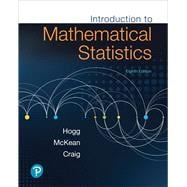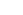# Introduction to Mathematical Statistics

• ISBN 13:

• ISBN 10:

## 0134686993

• Edition: 8th
• Format: Hardcover
• Publisher: Pearson

Note: Not guaranteed to come with supplemental materials (access cards, study guides, lab manuals, CDs, etc.)

Extend Your Rental at Any Time

Rental Options

List Price \$226.64 SaveFree Shipping On Every Order
Usually Ships in 7-10 Business Days

### Summary

For courses in mathematical statistics.

Comprehensive coverage of mathematical statistics — with a proven approach

Introduction to Mathematical Statistics by Hogg, McKean, and Craig enhances student comprehension and retention with numerous, illustrative examples and exercises. Classical statistical inference procedures in estimation and testing are explored extensively, and the text’s flexible organization makes it ideal for a range of mathematical statistics courses.

Substantial changes to the 8th Edition — many based on user feedback — help students appreciate the connection between statistical theory and statistical practice, while other changes enhance the development and discussion of the statistical theory presented.

0134686993 / 9780134686998   Introduction to Mathematical Statistics, 8/e